085-1263404

### My Journal

All things Mathematical
1 Oct 2018
##### Question 1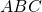is a steel rod, the portion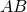being straight and of length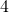feet and the portion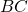being curved. The whole rod lies in one plane. The end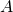is hinged to the floor and a load of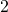cwt. is suspended from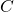by a rope which is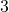feet fromwhich is kept vertical by applying a horizontal force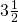feet from. The eight of the whole rod may be taken to becwt. acting at a distance of one foot fromwhen the latter is in the vertical position. Find the horizontal force and the reaction at the hinge in magnitude and direction.

##### Question 2is a trapezium,being parallel to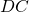.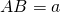,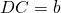. Show that the centroid of the area is one the line joining the mid-points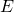,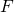of,and that it divides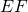in the ratio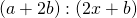.

##### Question 3

It is found that when a plane is inclined at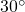to the horizontal a block of iron weighing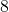lb. slides uniformly down the plane. Show that the angle of friction is.

When the plane is inclined at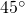to the horizontal the block is dragged slowly up the plane by a cord inclined at an angle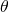to the plane. By means of the triangle of forces, or otherwise, find the tension in the cord and show that the tension is least when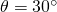.

##### Question 4

A wheel of radiusfeet is rolling, without slipping, on a horizontal plane ; the centre moving a uniform velocity of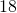feet per second. What is the angular velocity of the wheel?

What is the velocity of the extremity of the radius which is inclined atto the upward vertical through the centre of the wheel, the angle being measured in the direction of rotation of the wheel?

##### Question 5

Alb. weight is drawn up a rough plane, coefficient of friction, inclined atto the horizontal by a string, passing over a light smooth pulley at the top of the plane, to which is attached anlb. weight. Find the acceleration.

Calculate the kinetic energy when the system has moved a distance offt. and show that it is equal to the loss in potential energy minus the work done against friction.

##### Question 6

A car weighing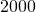pounds starts under the action of a force which decreases uniformly from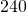lb. wt. at time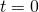to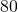lb. wt. at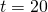seconds when it remains constant. The resistances to motion remain constant throughout and are equal tolb. wt. Show that the accelerating force at time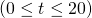is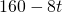lb. wt.

Find (a) the velocity at timesec.; (b) the distance travelled at timesec.; (c) the horsepower required when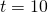sec. and also when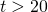secs.

##### Question 7

A gun fires a projectile with initial velocity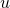and elevation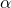. Show that (a) the range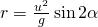; (b) the greatest height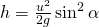, neglecting air resistance.

Show that the maximum range which can be obtained with the same initial velocity is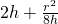.

##### Question 8

A cyclist is travelling round a curve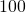feet radius at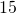miles per hour. At what angle must the track be inclined radially so that there may be no tendency to slip?

If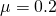what is the maximum speed on a level track of the same radius so that skidding may not occur? (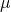is the coefficient of friction).

##### Question 9

If the period of a simple harmonic vibration is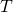seconds and the amplitude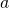feet, show that the maximum acceleration is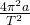.

A boy weighingstone standing on a plank oscillates vertically in simple harmonic motion of amplitude of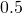foot and periodsecond. Find the greatest and least reactions of the plank.

##### Citation:

State Examinations Commission (2018). State Examination Commission. Accessed at: https://www.examinations.ie/?l=en&mc=au&sc=ru

Malone, D and Murray, H. (2016). Archive of Maths State Exams Papers. Accessed at: http://archive.maths.nuim.ie/staff/dmalone/StateExamPapers/

##### Licence:

“Contains Irish Public Sector Information licensed under a Creative Commons Attribution 4.0 International (CC BY 4.0) licence”.

The EU Directive 2003/98/EC on the re-use of public sector information, its amendment EU Directive 2013/37/EC, its transposed Irish Statutory Instruments S.I. No. 279/2005, S.I No. 103/2008, and S.I. No. 525/2015, and related Circulars issued by the Department of Finance (Circular 32/05), and Department of Public Expenditure and Reform (Circular 16/15 and Circular 12/16).

Note. Circular 12/2016: Licence for Re-Use of Public Sector Information adopts CC-BY as the standard PSI licence, and notes that the open standard licence identified in this Circular supersedes PSI General Licence No: 2005/08/01.